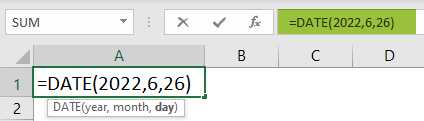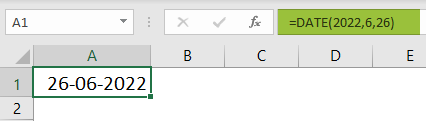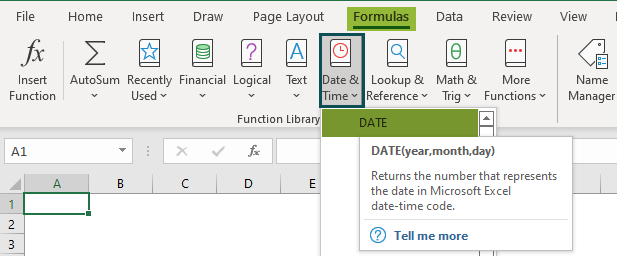# DATE Excel FunctionArticle byExcelMojo Team## What Is DATE Function In Excel?

The DATE function is used to enumerate dates in Excel. In simple terms, it combines Day, Month, and Year to form a Date. The DATE Excel formula is categorized under the Date & Time function in the Function Library.

For example, let us consider the following example where we need to format the date. We will learn how to use Date function in Excel.

Step 1: Enter the formula = DATE(2022,5,24) in cell A1.

Step 2: Press Enter key. We can see that the Date Excel function has returned the value in the format, as shown in the image below.

###### Key Takeaways
• The DATE function in Excel calculates the day, month, and year. It is a part of the Date & Time function of the Function Library.
• The formula of DATE function is =DATE(year,month,day) where
• year: It includes one to four digits.
• month: It can be a positive or negative number representing the month from 1 to 12.
• day: The day’s value can be positive or negative and denotes the day from 1 to 31.
• All three arguments are mandatory.
• The connected functions to the DATE function are DATEVALUE and EDATE functions.

### Date( ) Excel Formula

The syntax of the DATE Excel Function is

Unlike other functions, all three arguments are mandatory. The arguments are:

• year: The year argument includes one to four digits.
• month: It can be a positive or negative number representing the month from 1 to 12.
• day: The day’s value can be positive or negative and denotes the day from 1 to 31.

### How To Use Date Function? (With Steps)

We can insert Date function in excel using any of the following methods:

1. Access Date function from the Excel ribbon

1. Go to the Formulas tab of Excel.

2. Click on the Function Library

3. Choose the DATE function from the drop-down list of Date & Time

4. The Function Arguments window pops up.

5. Enter the arguments in the Year, Month, & Day

6. Click OK.

2. Enter the worksheet manually

1. Select an empty cell for the output.
2. Type =DATE( in the selected cell. Alternatively, type =D and double-click the DATE function from the list of suggestions shown by Excel.
3. Enter the arguments as Excel cell references or direct values.
4. Close the parenthesis and press the Enter key.

The below table shows the year, month, and day in columns A, B, C, and D, respectively. We need to find the date using the DATE function of Excel.

The steps to find the date are as follows:

Step 1:  Select an empty column for the output. We have selected column D in this case.

Step 2:  Next, start by entering the formula in cell D2.

Step 3: Select the cell that contains the year, i.e., A2.

Step 4: Next, choose the cell that contains the month, i.e., B2.

Step 5: Select the cell that contains the day, i.e., C2.

Thus, the complete formula is =DATE(A2,B2,C2).

Step 6:  Press Enter key.

Excel returns the date in cell D2 in the format Date-Month-Year (DD-MM-YYYY).

Step 7: Now, we can either drag the formula downwards till cell D3 using the AutoFill option or manually type the formula and select the arguments’ values.

In our example, let us use the AutoFill option.

We can see that excel has used the values and returned them in the date format.

### Examples

Let us learn how to use Date function in Excel with the following examples.

#### Example 1 – Using DATEVALUE

The DATEVALUE function converts the data format into the serial number it represents.

The formula of the DATEVALUE function is;

=DATEVALUE(date_text)

Let us understand this function better with the help of an example.

For example, the below table shows one date in two different formats. We need to use the following steps to calculate the date value using the DATEVALUE function of Excel.

The steps to find the date value are as follows:

Step 1:  Select an empty column for the output. We have selected column B in this case.

Step 2:  Next, start by entering the formula in cell B2.

Select cell A2 as the argument.

So, the complete formula is =DATEVALUE(“24-May-2022”).

Step 3: Press the Enter key. We can see that the function has returned the numeric value of the date we entered in the formula.

Step 4: We can drag the formula downwards using the AutoFill option till cell B3.

Irrespective of the format, Excel returns the same numeric value.

#### Example 2 – Using EDATE

The EDATE function returns the date’s serial number, the specified number of months before or after the start date.

The formula of the EDATE function is;

=EDATE(start_date,months)

Let us understand better with the help of an example.

For example, the below table shows the date in column A. We need to calculate the date after the month’s value using the EDATE function of Excel.

The steps to calculate the date are as follows:

Step 1:  Select an empty cell for the output. We have selected cell B2 in this case.

Step 2:  Next, start by entering the formula in cell B2.

Step 3: Select the cell that contains the start date, i.e., ‘A2.’

Step 4: Enter the months we want to calculate the date, i.e., ‘2.’

The complete formula is =EDATE(A2,2).

Step 5:  Press the Enter key. The image below shows the results in cell B2 as 24 July 2022.

#### Example – 3 – Using DATE function extract Month

The following table shows the date in column A. We need to use the below steps to calculate the month using the MONTH function with the DATE function of Excel.

The steps to calculate date are as follows:

Step 1:  Select an empty column for the output. We have selected column B in this case.

Step 2: Next, start by entering the formula in cell B2.

Step 3: The complete formula is =MONTH(DATE(2022,5,1)).

Step 4: Press the Enter key. The results are shown in cell B2 as ‘5’ as shown in the image below.

Step 5: Press the Enter key. Drag the formula downwards till cell B3.

#### Example – 4 – Using DATE function, find the leap year

The below table shows the dates in column A. We need to check whether the given year is a leap year or not using the DATE formula with the MONTH and YEAR formula.

The steps to calculate the Date are as follows:

Step 1:  Select an empty column for the output. We have selected column B in this case.

Step 2:  Next, start by entering the formula in cell B2.

Step 3: The complete formula is =MONTH(DATE(YEAR(A2),2,29))=2.

Step 4: Press the Enter key. The results are shown in cell B2 as ‘TRUE’ since 2020 is a leap year.

Step 5: Press the Enter key. Drag the formula downwards till cell B3.

Hence, the result is returned as “TRUE” for 2020 and “FALSE” for 2022.

Please Note: We can test if it is a leap year or not. But since, February has 29 days in leap years, the DATE function will return the output as 2/29/2020.

In the case of a non-leap year, DATE function will return 1st March because there are only 28 days. So, the DATE would roll the date forward into the next month.

### Important Things To Note

• The DATE function in Excel returns a date serial number. We must format the result as a date to display the date format.
• The Excel will add 1900 to the year to display as a return.
• We can create a list of sequential dates.
• We can also calculate the number of days between two dates by subtracting them.
• #NUM! error occurs when the argument is less than 0 or greater than or equal to 10000.
• #VALUE! error occurs when the argument passed is non-numeric.

What does DATE Function do in Excel?

The DATE function combines the three components as Day, Month, & Year and returns the Date in a format.

The DATE function formula is =DATE(year,month,day)

How does the DATE format work in Excel?

The steps used to format the date in excel are as follows:

1. Select the cell to display the output.
2. Type the formula =DATE(
3. Select the year, month, and day as arguments.
4. Press Enter

For example, consider the following example where we need to format the date. Let us learn how to use date function in excel.

Step 1:  Enter the formula = DATE(2022,5,26) in cell A1.Step 2:  Press Enter key.

We can see that the Date excel function has returned the value in the format, as shown in the image below.Thus, we can format dates using date function in excel.

Where is the DATE Function in Excel?

The DATE Excel Function is available by default. We can use it by following the below steps:

1. Select the cell which has the number.
2. Select the Formulas tab.
3. Click on the Date & Time option.
4. Select the DATE option from the drop-down list.
5. The Function Arguments window pops up.
6. Enter the value in the Year, Month, and Day as the number of arguments.
7. Click OK.